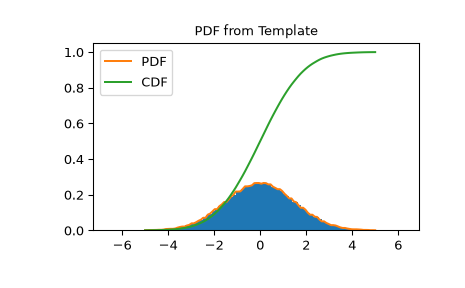# scipy.stats.rv_histogram¶

class scipy.stats.rv_histogram(histogram, *args, **kwargs)[source]

Generates a distribution given by a histogram. This is useful to generate a template distribution from a binned datasample.

As a subclass of the `rv_continuous` class, `rv_histogram` inherits from it a collection of generic methods (see `rv_continuous` for the full list), and implements them based on the properties of the provided binned datasample.

Parameters
histogramtuple of array_like

Tuple containing two array_like objects The first containing the content of n bins The second containing the (n+1) bin boundaries In particular the return value np.histogram is accepted

Notes

There are no additional shape parameters except for the loc and scale. The pdf is defined as a stepwise function from the provided histogram The cdf is a linear interpolation of the pdf.

New in version 0.19.0.

Examples

Create a scipy.stats distribution from a numpy histogram

```>>> import scipy.stats
>>> import numpy as np
>>> data = scipy.stats.norm.rvs(size=100000, loc=0, scale=1.5, random_state=123)
>>> hist = np.histogram(data, bins=100)
>>> hist_dist = scipy.stats.rv_histogram(hist)
```

Behaves like an ordinary scipy rv_continuous distribution

```>>> hist_dist.pdf(1.0)
0.20538577847618705
>>> hist_dist.cdf(2.0)
0.90818568543056499
```

PDF is zero above (below) the highest (lowest) bin of the histogram, defined by the max (min) of the original dataset

```>>> hist_dist.pdf(np.max(data))
0.0
>>> hist_dist.cdf(np.max(data))
1.0
>>> hist_dist.pdf(np.min(data))
7.7591907244498314e-05
>>> hist_dist.cdf(np.min(data))
0.0
```

PDF and CDF follow the histogram

```>>> import matplotlib.pyplot as plt
>>> X = np.linspace(-5.0, 5.0, 100)
>>> plt.title("PDF from Template")
>>> plt.hist(data, density=True, bins=100)
>>> plt.plot(X, hist_dist.pdf(X), label='PDF')
>>> plt.plot(X, hist_dist.cdf(X), label='CDF')
>>> plt.show()
```Attributes
`random_state`

Get or set the generator object for generating random variates.

Methods

 `__call__`(*args, **kwds) Freeze the distribution for the given arguments. `cdf`(x, *args, **kwds) Cumulative distribution function of the given RV. `entropy`(*args, **kwds) Differential entropy of the RV. `expect`([func, args, loc, scale, lb, ub, ...]) Calculate expected value of a function with respect to the distribution by numerical integration. `fit`(data, *args, **kwds) Return estimates of shape (if applicable), location, and scale parameters from data. `fit_loc_scale`(data, *args) Estimate loc and scale parameters from data using 1st and 2nd moments. `freeze`(*args, **kwds) Freeze the distribution for the given arguments. `interval`(alpha, *args, **kwds) Confidence interval with equal areas around the median. `isf`(q, *args, **kwds) Inverse survival function (inverse of `sf`) at q of the given RV. `logcdf`(x, *args, **kwds) Log of the cumulative distribution function at x of the given RV. `logpdf`(x, *args, **kwds) Log of the probability density function at x of the given RV. `logsf`(x, *args, **kwds) Log of the survival function of the given RV. `mean`(*args, **kwds) Mean of the distribution. `median`(*args, **kwds) Median of the distribution. `moment`(n, *args, **kwds) n-th order non-central moment of distribution. `nnlf`(theta, x) Negative loglikelihood function. `pdf`(x, *args, **kwds) Probability density function at x of the given RV. `ppf`(q, *args, **kwds) Percent point function (inverse of `cdf`) at q of the given RV. `rvs`(*args, **kwds) Random variates of given type. `sf`(x, *args, **kwds) Survival function (1 - `cdf`) at x of the given RV. `stats`(*args, **kwds) Some statistics of the given RV. `std`(*args, **kwds) Standard deviation of the distribution. `support`(*args, **kwargs) Support of the distribution. `var`(*args, **kwds) Variance of the distribution.